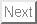Book
Tetsushi Ueta, Hiroshi Kawakami, Numerical approaches to bifurcation analysis, G. Chen and T. Ueta (eds), in Chaos in Circuits and Systems, Chapter 28World Scientific, 593-610, Singapore, July 2002.
Abstract: This chapter investigates direct methods for evaluating the bifurcation parameter values of periodic solutions in nonlinear systems. The method can numerically calculate parameter values with high convergence rate and accuracy, at which local bifurcations; tangent, period-doubling, Neimark-Sacker and pitchfork bifurcations are occurred. The method uses some basic properties of the characteristic equation for a fixed point of the corresponding Poincare map. The location and parameter value of the fixed point are used as independent variables in the computation that employs quadratically convergency in Newton method. In addition, in the case of Neimark-Sacker bifurcation, the argument of the complex conjugate multiplier or the other parameter is used as the independent variable. This direct method utilize accurate solution of variational equations with numerical integration, thus implementaion of algorithm becomes very simple. Some numerical examples are given.